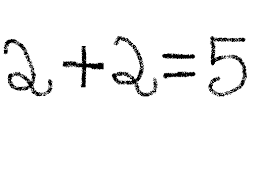# You must be crazy!Why? What’s wrong with that…?

Teachers and tutors ask pupils to check their work, but how can you do that in Maths without doing the whole sum all over again? Well, you can’t! So how are you supposed to check your work?

What you have to understand first of all is that checking everything is right is very different from checking nothing is obviously wrong. To check everything is right means doing the whole paper twice, but you obviously don’t have time to do that. Checking nothing is obviously wrong is much easier because it just means doing a ‘quick and dirty’ calculation in your head. It doesn’t guarantee that the answer is right, but it’s a good compromise. I call it ‘sanity checking’, which means making sure your answers are not crazy! Unfortunately, there isn’t one method that works for every question – it depends on what type of question it is – but here are a few examples:

Algebra

If you have to ‘solve for 𝑥’ and it’s a difficult question, try putting your answer back into the original equation and seeing if one side equals the other, eg if you think 𝑥 = 5, then that works for 2𝑥 + 6 = 16, but not for 3𝑥 + 2 = 5. That would be crazy!

Multiplication

Every multiplication sum starts with multiplying the last digit of each number together, so try doing that when you’ve got your answer and checking if the last digit of the result is equal to the last digit of the answer, eg 176 x 467 is going to end in a 2 because 6 x 7 = 42, which also ends with a 2. Your answer couldn’t end in any other number. That would be crazy!

Rounding

If you have any kind of sum that involves adding, subtracting, multiplication or division, an easy way to check it is to round the numbers to one or two significant figures (eg to the nearest hundred) and work out the answer in your head. If it’s close enough, then your answer is not obviously wrong. If it’s nowhere near, then you’ll have to do it again, eg 1.7 x 3.4 is close to 2 x 3, so the answer might be 5.78, but it wouldn’t be 57.8. That would be crazy!

Units

Most answers in Maths tests need some kind of unit, such as kg, m, cm or ml. Sometimes, the units are provided, but sometimes they’re not. If they’re not, you just need to make sure that you use the right ones, eg if the scale of a map is 1:100,000, the distance represented by 9.8cm is 9.8km, not 9.8m. That would be crazy!